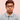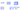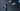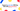# S Y

Navigate back to the homepage
Subscribe

# DataStructure and Algorithm Series in JavaScriptMay 11th, 2021 · 8 min readWith this post I am starting a new series where I will cover all major DataSctructure and Algorithms in an easy and illustrative manner.

The terms in table of content might seem very scary, but just be with me I promise to explain everything in the simplest manner possible.

I will keep updating this post on a daily basis, till I cover everything mentioned in Table of content.

## Tabel of content:

• Big O Notation
• Understanding Big O Notation
• Algorithms
• DataStructure

## Understanding Big O Notation

Big O Notation is a way to represent the time and space complexity of an algorithm.

• Time Complexity: Time taken by the algorithm to complete the execution.
• Space Complexity: The memory occupied by the algorithm.There are few expressions (notations) which represents the time complexity of an algorithm.

• O(1): Constant time complexity. This is the ideal case.
• O(log n): Logarithmic time complexity. If `log(n) = x` then it is same as `10^x`
• O(n): Linear time complexity. Time increases with the number of input in a linear manner. For ex, If one input takes 1 ms, 4 inputs will take 4 ms to execute the algo.
• O(n^2): Exponential time complexity. This mostly happens in case of nested loops.
• O(n!): Factorial time complexity. This is the worst case senario, which should be avoided.

You should try to write your algorithm such that it can be represented by the first 3 notations. And last two should be avoided as often as possible.You want to keep your complexity as low and straight as possible, ideally avoiding anything above O(n).

In further sections of this article you will see examples of each notation. For now this is all you need to know.

## Algorithm

### What is algorithm and why to care?

The way to solve a problem or we can say the steps, procedure, or set of rules to solve a problem is known as Algorithm.

ex: Search Engine algorithm to find out data related to a search string.

As a programmer you will come across many problems that needs to be solved with these algorithms. So, it’s better if you already know them.

### Recursion

A function calling itself is recursion. Think of it as an alternative to loop.

`.css-1chxjt6{position:absolute;right:22px;top:24px;padding:8px 12px 7px;border-radius:5px;color:#6f7177;-webkit-transition:background 0.3s ease;transition:background 0.3s ease;}.css-1chxjt6:hover{background:rgba(255,255,255,0.07);}.css-1chxjt6[data-a11y="true"]:focus::after{content:"";position:absolute;left:-2%;top:-2%;width:104%;height:104%;border:2px solid var(--theme-ui-colors-accent,#6166DC);border-radius:5px;background:rgba(255,255,255,0.01);}@media (max-width:45.9375em){.css-1chxjt6{display:none;}}1function recursiveFn() {2    console.log("This is a recursive function");3    recursiveFn();4}56recursiveFn();`

In the above snippet look at line 3 recursiveFn is called in recursiveFn itself. As I mentioned earlier recursion is an alternative to loop.

So, how many times this function is exactly going to run?

Well, this will create an infinite loop, because there is nothing to stop it at any point.Let’s say we need to run the loop only 10 times. On the 11th iteration function should return. That will stop the loop.

`1let count = 1;2function recursiveFn() {3    console.log(`Recursive \${count}`);4    if (count === 10) return;5    count++;6    recursiveFn();7}89recursiveFn();`

In the above snippet line 4 returns and stops the loop at count 10.

Now let’s see a more realistic example. Our task is to return an array of odd numbers from a given array. This can be achieved in a number of ways including for-loop, Array.filter method, e.t.c

But to showcase the use of recursion I will use a helperRecursive function.

`1function oddArray(arr) {2    let result = [];3    function helperRecursiveFn(arr) {4        if(arr.length === 0) {5            return; // 16        } else if(arr % 2 !== 0) {7            result.push(arr); // 28        }9        helperRecursiveFn(arr.slice(1)); // 310    }11    helperRecursiveFn(arr);12    return result;13}1415oddArray([1, 2, 3, 4, 5, 6, 7, 8, 9, 10]);16// OutPut -> [1, 3, 5, 7, 9]`

Here the recursive function is helperRecursiveFn.

1. Return if the array length is 0.
2. Push the element to result array if the element is odd.
3. Call helperRecursiveFn with first element of array sliced. Every time the first element of array will be sliced, because we have already cheked it for odd or even.

For ex: First time helperRecursiveFn will be called with `[1, 2, 3, 4, 5, 6, 7, 8, 9, 10]`. Next time it will be called with `[2, 3, 4, 5, 6, 7, 8, 9, 10]` and so on untill the array length is 0.

### Linear Search Algorithm

Linear search algorithm is pretty simple. Say that you need to find if a number exists in a given array or not.

You will run a simple for loop and check every element untill you find the one you are looking for.

`1const array = [3, 8, 12, 6, 10, 2];23// Find 10 in the given array.4function checkForN(arr, n) {5    for(let i = 0; i < array.length; i++) {6        if (n === array[i]) {7            return `\${true} \${n} exists at index \${i}`;8        }9    }1011  return `\${false} \${n} does not exist in the given array.`;12}1314checkForN(array, 10);`

That’s linear search algorithm. You search for each element in the array one by one in a linear manner.#### Time Complexity of Linear Search Algorithm

There is only one for loop which will run n times. Where n (in worst case) is the length of the given array. Here number of iterations (in worst case) is directly propotional to the input (array of length).

Hence the time complexity for linear search algorithm is Linear Time Complexity: O(n).

### Binary Search Algorithm

In linear search you can eliminate one element at a time. But with binary search algorithm you can eliminate multiple elements at once. That’s why binary search is faster than linear search.

The point to be noted here is that binary search works only on sorted array.

This algorithm follows divide and conquer approach. Let find the index of 8 in [2, 3, 6, 8, 10, 12].

Step 1: Find the middleIndex of the array.

`1const array = [2, 3, 6, 8, 10, 12];2let firstIndex = 0;3let lastIndex = array.length - 1;4let middleIndex = Math.floor((firstIndex + lastIndex) / 2); // middleIndex -> 2`

Step 2: Check if middleIndex element > 8. If so, that means 8 is on the left of middleIndex. Hence, change lastIndex to (middleIndex - 1).

Step 3: Else if middleIndex element < 8. That means 8 is on the right of middleIndex. Hence, change firstIndex to (middleIndex + 1);

`1if (array[middleIndex] > 8) {2    lastIndex = middleIndex - 1;3} else {4    firstIndex = middleIndex + 1;5}`

Step 4: With every iteration middleIndex is again set as per the new firstIndex or lastIndex.

Let’s see all those steps together in code format.

`1function binarySearch(array, element) {2    let firstIndex = 0;3    let lastIndex = array.length - 1;4    let middleIndex = Math.floor((firstIndex + lastIndex) / 2);5    6    while (array[middleIndex] !== element && firstIndex <= lastIndex) {7        if(array[middleIndex] > element) {8                lastIndex = middleIndex - 1;9        }else {10                firstIndex = middleIndex + 1;11        }12        middleIndex = Math.floor((firstIndex + lastIndex) / 2);13    }14    return array[middleIndex] === element ? middleIndex : -1;15}1617const array = [2, 3, 6, 8, 10, 12];18binarySearch(array, 8); // OutPut -> 3`

Here is visual representation of the above code.

Step: 1`1firstIndex = middleIndex + 1;`

Step: 2`1lastIndex = middleIndex - 1;`

Step: 3`1array[middleIndex] === 8 // Found It`

There is only one while loop which will run n times. But here number of iterations does not depend on the input (array length).

Hence the time complexity for binary search algorithm is Logarithmic Time Complexity: O(log n). And you can check the O-notation graph. O(log n) is faster than O(n).

### Naive Search Algorithm

Naive search algorithm is used to find if a string contains a given substring. For example, check if “helloworld” contains the substring “owo”.

1. First loop on the main string (“helloworld”).
2. Run a nested loop on the substring (“owo”).
3. If character does not match then break inner loop else keep looping.
4. If inner loop is completed and got a match, then return true else keep the outer loop going.

Here is a visual representation.Here is the implementation in code.

`1function naiveSearch(mainStr, subStr) {2    if (subStr.length > mainStr.length) return false;34    for(let i = 0; i < mainStr.length; i++) {5       for(let j = 0; j < subStr.length; j++) {6            if(mainStr[i + j] !== subStr[j]) break;7            if(j === subStr.length - 1) return true; 8        }9    }10    return false;11}`

Now, let’s try to understand the code above.

• At line 2, return false if subString length is greater than mainString length.
• At line 4, start looping on mainString.
• At line 5, start nested loop on subString.
• At line 6, break inner loop if no match is found, And move on to next iteration for outer loop.
• At line 7, return true at last iteration of inner loop.

There is a loop inside a loop (Nested Loop). Both loop run n times. Hence time complexity for naive search algo is (n * n) Exponential Time Complexity: O(n^2).

And as discussed at top, any time complexity above O(n) should be avoided if possible. We will see a better approach with less time complexity in next algo.

### KMP Algorithm

KMP algo is a pattern recongnition algorithm, and it is a bit tough to understand. Ok, let’s try to find if the string “abcabcabspl” contains the sub string “abcabs”.

If we try to solve this with Naive Search Algo, it will match for first 5 characters but not for 6th character. And we will have to start over again with next iteration, we will lost all the progress in previous iteration.So, in order to save our progress and use it, we must use something called LPS table. Now in our matched string “abcab” we will find the longest same prefix and suffix.

Here, in our string “abcab” “ab” is the longest same prefix and suffix.Now, we will begin the next search iteration from index 5 (for main string). We saved two characters from our previous iteration.In order to figure out the prefix, suffix, and where to start next iteration from we use LPS table.

LPS for our substring (“abcabs”) is “0 0 0 1 2 0”.Here is how to calculate LPS table.

`1function calculateLpsTable(subStr) {2    let i = 1;3    let j = 0;4    let lps = new Array(subStr.length).fill(0);56    while(i < subStr.length) {7        if(subStr[i] === subStr[j]) {8            lps[i] = j + i;9            i += 1;10            j += 1;11        } else {12            if(j !== 0) {13                j = lps[j - 1];14            } else {15                i += 1;16            }17        }18    }19    return lps;20}`

Here is the implementation in code using LPS table.

`1function searchSubString(string, subString) {2    let strLength = string.length;3    let subStrLength = subString.length;4    const lps = calculateLpsTable(subString);56    let i = 0;7    let j = 0;89    while(i < strLength) {10        if (string[i] === subString[j]) {11            i += 1;12            j += 1;13        } else {14            if (j !== 0) {15                j = lps[j - 1];16            } else {17                i += 1;18            }19        }20        if (j === subStrLength) return true;21    }2223    return false;24}`

#### Time Complexity of KMP Algorithm

There is only one loop which run n times. Hence time complexity for KMP algo is Linear Time Complexity: O(n).

Notice how time complexity is improved as compared to that of Naive search algo.

### Bubble Sort Algorithm

Sorting means rearranging data in ascending or descending order. Bubble sort is one of many sorting algorithms.

In bubble sort algo, we swap the larger number to the end by comparing each number with the previous number. Here is a visual representation.Bubble sort code implementation.

`1function bubbleSort(array) {2    let isSwapped;34    for(let i = array.length; i > 0; i--) {5        isSwapped = false;67        for(let j = 0; j < i - 1; j++) {8            if(array[j] > array[j + 1]) {9                [array[j], array[j+1]] = [array[j+1], array[j]];10                isSwapped = true;11            }12        }1314        if(!isSwapped) {15            break;16        }17    }18    return array;19}`

Let’s try to understand the above code.

• Looping from end of array with variable i towards beginning.
• Start inner loop with variable j until (i - 1).
• If array[j] > array[j + 1] swap them.
• return sorted array.

#### Time Complexity of Bubble Sort Algorithm

There is a nested loop and both loop runs n times, so time complexity for this algo is (n * n) that is Exponential Time Complexity O(n^2).

### Merge Sort Algorithm

Merge sort algorithm follows divide and conquer approach. It’s a combination of two things - merge and sort.

In this algo first we divide main array into multiple indivisual sorted arrays.Then we merge the indivisual sorted elements together into final array.

Let’s look at the implementation in code.

Merge Sorted Array

`1function mergeSortedArray(array1, array2) {2    let result = [];3    let i = 0;4    let j = 0;56    while(i < array1.length && j < array2.length) {7        if(array1[i] < array2[j]) {8            result.push(array1[i]);9            i++;10        } else {11            result.push(array2[j]);12            j++;13        }14    }1516    while (i < array1.length) {17        result.push(array1[i]);18        i++;19    }2021    while (j < array2.length) {22        result.push(array2[j]);23        j++;24    }2526    return result;27}`

The above code merges two sorted array into a new sorted array.

Merge Sort Algorithm

`1function mergeSortedAlgo(array) {2    if(array.length <= 1) return array;34    let midPoint = Math.floor(array.length / 2);5    let leftArray = mergeSortedAlgo(array.slice(0, midPoint));6    let rightArray = mergeSortedAlgo(array.slice(midPoint));78    return mergeSortedArray(leftArray, rightArray);9}`

The above algo uses recursion to divide the array into multiple single element array.

#### Time Complexity of Merge Sort Algorithm

Let’s try to calculate time complexity of merge sort algorithm. So, taking our previous example ([6, 3, 5, 2]), it took 2 steps to divide it into multiple single element array.

`It took 2 steps to divide an array of length 4 - (2^2)`.

Now if we double the length of array (8), it will take 3 steps to divide - (2^3). Means doubling the array length didn’t doubled the steps.

Hence time complexity of merge sort algorithm is Logarithmic Time Complexity O(log n).

### Quick Sort Algorithm

Quick sort is one of the fastest sorting algorithm. In quick sort we select a single element known as pivot and we will move all element (smaller than pivot) to the left of pivot.

A visual representation.We will repeat this process for array to left and right of pivot until the array is sorted.

Code implementation

Pivot Utility

`1function pivotUtility(array, start=0, end=array.length - 1) {2    let pivotIndex = start;3    let pivot = array[start];4    5    for(let i = start + 1; i < array.length; i++) {6        if(pivot > array[i]) {7            pivotIndex++;8            [array[pivotIndex], array[i]] = [array[i], array[pivotIndex]];9        }   10    }1112    [array[pivotIndex], array[start]] = [array[start], array[pivotIndex]];13    return pivotIndex;14}`

The above code identifies the correct position of pivot and returns that position index.

`1function quickSort(array, left=0, right=array.length-1) {2    if (left < right) {3        let pivotIndex = pivotUtility(array, left, right);4        quickSort(array, left, pivotIndex - 1);5        quickSort(array, pivotIndex + 1, right);6    }78    return array;9}`

The above code uses recursion to keep moving pivot to it’s correct position for left and right array of pivot.

#### Time Complexity of Quick Sort Algorithm

BEST CASE: Logarithmic Time Complexity - O(n log n)
AVERAGE CASE: Logarithmic Time Complexity - O(n log n)
WORST CASE: O(n^2)Radix sort is also know as Bucket sort algorithm.

Here first we build 10 buckets of index from 0 to 9. Then we take last character in each number and push the number to the corresponding bucket. Retrive the new order and repeat for the second last character of each number.

Keep repeating the above process till the array is sorted.

Implementation in code.

// Count Digits: The code below counts the number of digits the given element have.

`1function countDigits(number) {2    if(number === 0) return 1;3    4    return Math.floor(Math.log10(Math.abs(number))) + 1;5}`

// Get Digit: The code below gives the digit at index i from right.

`1function getDigit(number, index) {2    const stringNumber = Math.abs(number).toString();3    const currentIndex = stringNumber.length - 1 - index;45    return stringNumber[currentIndex] ? parseInt(stringNumber[currentIndex]) : 0;6}`

// MaxDigit: The below snippet finds the number with maximum digits.

`1function maxDigit(array) {2    let maxNumber = 0;34    for(let i = 0; i < array.length; i++) {5        maxNumber = Math.max(maxNumber, countDigits(array[i]));6    }7    8    return maxNumber;9}`

// The Radix Algo: Utilises all the above snippets to sort the array.

`1function radixSort(array) {2    let maxDigitCount = maxDigits(array);34    for(let i = 0; i < maxDigitCount; i++) {5        let digitBucket = Array.from({length: 10}, () => []);67        for(let j = 0; j < array.length; j++) {8            let lastDigit = getDigit(array[j], i);9            digitBucket[lastDigit].push(array[j]);10        }1112        array = [].concat(...digitBucket);13    }1415    return array;16}`

#### Time complexity of Radix Sort Algorithm

There is a nested for loop, and we know that time complexity for a nested for loop is O(n^2). But in this case both for loop does not run n times.

The outer loop runs k (maxDigitCount) times and inner loop runs m (length of array) times. Hence time complexity of Radix Sort is O(k x m) - (where k x m = n) Linear time complexity O(n)

## DataStructure

### What is DataStructure and why to care?

DataStructure is the way to organize your data. Different operations (CRUD) performs better with different DataStructures.

So, it is very important to know which DS is right for a particular use case.

I am updating this post daily with one DS / Algo topic. If this is helpful to you in any way, consider sharing with your social media network.

Thank You!

### More articles from Swastik Yadav### My first developer job and why I left it

How I got my first dev job as a college drop out without a CS degree and why I left it.

April 27th, 2021 · 3 min read### Convert px to rem - An effective workflow

Why you should convert all your px to rem to have an adaptable and responsive web page out of the box.

April 24th, 2021 · 2 min read

### Become a better full-stack developer in 10 minutes a week.

Be the first to receive our latest content with the ability to opt-out at anytime. We promise to not spam your inbox or share your email with any third parties.